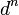# Binomial formula for powers of a derivation

## Statement

Suppose$R$ is a Non-associative ring (?) (i.e., a not necessarily associative ring -- this includes the associative ring, Lie ring, and other cases) and$d$ is a derivation of$R$. For any nonnegative integer$n$, if we denote by$d^n$ the composition of$d$ with itself$n$ times, with$d^0$ defined as the identity map.

We then have:$\! d^n(x * y) = \sum_{i=0}^n \binom{n}{i} \left(d^i(x) * d^{n-i}(y)\right)$

Note that this in particular applies to the case of a Derivation of a Lie ring (?) and a Derivation of an associative ring (?).

## Proof

### Proof idea

The idea is to use the Leibniz rule (which establishes the case$n = 1$;$n = 0$ is vacuous) and prove by induction. The proof is almost exactly like the proof of the binomial formula for$(x + y)^n$ for a commutative associative algebra.

### Proof details: case$n = 0$

In the case$n = 0$, both sides are$x * y$, so the equality holds vacuously.

### Proof details: case$n = 1$

In this case, the left side is$d(x * y)$. The right side is:$\! \binom{1}{0} (d^0(x) * d^1(y)) + \binom{1}{1}(d^{1}(x) * d^{0}(y)) = (x * dy) + (dx * y)$

By the Leibniz rule, the left side equals the right side.

### Proof details: inductive step

Inductive hypothesis:$\! d^{n-1}(x * y) = \sum_{i=0}^{n-1} \binom{n - 1}{i} d^i(x) * d^{n-1-i}(y)$

To prove:$\! d^{n}(x * y) = \sum_{i=0}^{n} \binom{n}{i} d^i(x) * d^{n-1-i}(y)$

Proof: We have by definition:$\! d^n(x * y) = d\left(d^{n-1}(x * y)\right)$

By the inductive hypothesis, we can expand the inside and we get:$\! d^n(x * y) = d\left\{\sum_{i=0}^{n-1} \binom{n - 1}{i} d^i(x) * d^{n-1-i}(y)\right\}$

Since$d$ is additive, we can pull it inside the summation on the right side and get:$\! d^n(x * y) = \sum_{i=0}^{n-1}\binom{n - 1}{i}d\left\{d^i(x) * d^{n-1-i}(y)\right\}$

Now using the Leibniz rule on the inside, we get:$\! d^n(x * y) = \sum_{i=0}^{n-1}\binom{n - 1}{i}\left\{(d^{i+1}(x) * d^{n-(i+1)}(y)) + (d^i(x) * d^{n-i}(y))\right\}$

We now rearrange the summation and obtain:$\! d^n(x * y) = \sum_{i=0}^n \left(\binom{n - 1}{i} + \binom{n - 1}{i-1}\right)(d^i(x) * d^{n-i}(y))$

We use Pascal's identity on the sum of binomial coefficients and obtain what we want:$\! d^{n}(x * y) = \sum_{i=0}^{n} \binom{n}{i} d^i(x) * d^{n-1-i}(y)$